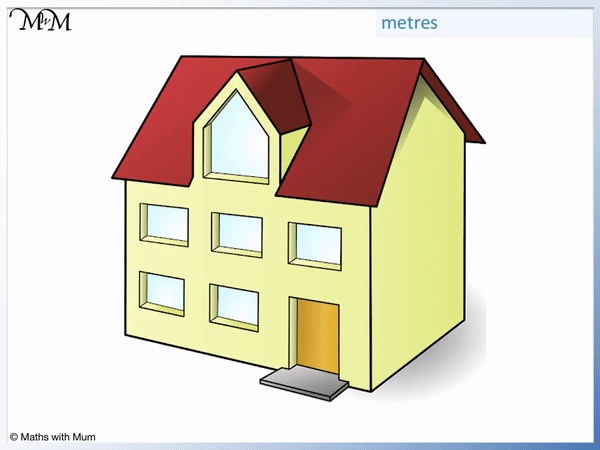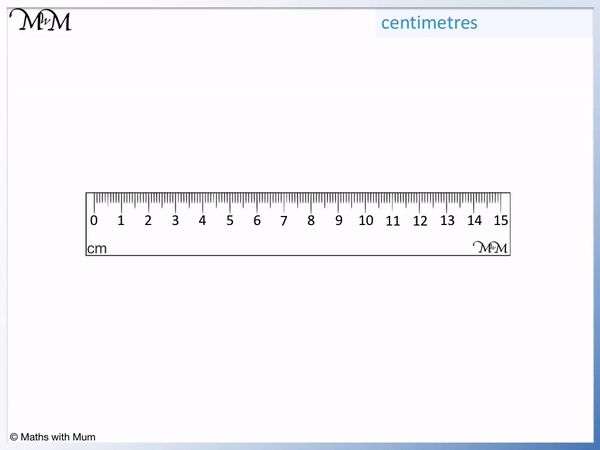# Units of Length

Units of Length• A millimetre is the smallest measurement on a ruler. A millimetre is about as thick as a staple or the tip of a pencil.
• Millimetres are usually used to measure objects that can be held in one hand. They can also be used to measure larger objects that need greater accuracy, such as the size of a phone screen.
• There are 10 millimetres in a centimetre.
• Centimetres are usually used to measure objects that are smaller than a ruler. They can also be used to measure larger objects with greater accuracy, such as a person’s height.
• There are 100 centimetres in a metre.
• Metres are usually used to measure objects that are longer than the width of a doorway but they can be used to measure smaller objects too.
• There are 1000 metres in a kilometre.
• Kilometres are usually used to measure distances between places such as towns, cities and countries as well as the length of roads.
The most common metric units of length are kilometres (km), metres (m), centimetres (cm) and millimetres (mm).

There are 10 mm in 1 cm, 100 cm in 1 m and 1000 m in 1 km.

• The common imperial units of length are inches (in), feet (ft), yards (yd) and miles (mi).
• These units of length are most commonly used in the USA.
• There are 12 inches in a foot.
• There are 3 feet in a yard
• There are 1760 yards in a mile.• There are 10 millimetres in every centimetre.
• 8 cm can be written in millimetres by multiplying it by 10.
• 8 x 10 = 80 and so, 8 cm = 80 mm.# Units of Length

## Metric Units of Length

From smallest to largest, the metric units of length are millimetres (mm), centimetres (cm), metres (m) and kilometres (km). There are 10 mm in 1 cm, 100 cm in 1 m and 1000 m in 1 km. The metric units of length are used in all countries except the USA, Liberia and Myanmar.

Here is a table showing the most common metric units of length and how to convert between them.

To convert between metric units of length, use the following rules:

• To convert cm to mm, multiply by 10.
• To convert m to cm, multiply by 100.
• To convert km to m, multiply by 1000.
• To convert mm to cm, divide by 10.
• To convert cm to m, divide by 100.
• To convert m to km, divide by 1000.

Here are some examples of converting between metric units:

Original Metric Unit Conversion Equivalent Metric Unit
20 mm ÷ 10 2 cm
36 mm ÷ 10 3.6 cm
200 cm ÷ 100 2 m
518 cm ÷ 100 5.18 m
5000 m ÷ 1000 5 km
7200 m ÷ 1000 7.2 km
8 km × 1000 8000 m
6.3 km × 1000 6300 m
5 m × 100 500 cm
3.4 m × 100 340 cm
9 cm × 10 90 mm
6.5 cm × 10 65 cm
3 km × 100 000 300 000 cm
2.5 m × 1000 2500 mm

## Imperial Units of Length

From smallest to largest, the most common imperial units of length are inches (in), yards (yd), feet (ft) and miles (mi). There are 12 inches in a foot, 3 feet in a yard and 1760 yards in a mile. Imperial units of length are used in the USA, Liberia and Myanmar.

Here is a table showing the most common imperial units of length and how to convert between them.

To convert between metric units of length, use the following rules:

• To convert feet to inches, multiply by 12.
• To convert yards to feet, multiply by 3.
• To convert miles to yards, multiply by 1760.
• To convert inches to feet, divide by 12.
• To convert feet to yards, divide by 3.
• To convert yards to miles, divide by 1760.

## What is the Difference Between Metric and Imperial Units?

Metric units use base 10 conversions, whereas imperial units do not. This means that it is easier to convert between metric units by multiplying or dividing by 10, 100 or 1000.

Metric units have a consistent conversion ratio across different measurements whereas imperial units do not. This makes it easier to remember and work with metric units. For example, to convert from kilometres to metres, we multiply by 1000 and to convert from kilograms to grams, we also multiply by 1000. We do not have similar patterns with imperial units such as inches to feet or ounces to pounds, which can make it more challenging to work with these units.

Metric units are used consistently in most countries throughout the world. Only America, Libera and Myanmar officially use imperial units. These countries still use these traditional imperial units because they have been working with imperial units for many years and it would be costly to convert everything into metric units.

Although similar in size, a metre is not the same length as a yard. 1 m is equal to 1.09 yards and so, 1 metre is longer than 1 yard by approximately 3 inches or 8 centimetres.

A centimetre is smaller than an inch. A centimetre is less than half the size of an inch. 1 inch is approximately equal to 2.5 centimetres.

## Units of Length List

The most common units for length are shown in the list below with examples:

Metric Units of Length Example Conversion
1 millimetre (mm) Thickness of a staple 10 mm = 1 cm
1 centimetre (cm) The width of a little finger 100 cm = 1 m
1 metre (m) The width of a doorway 1000 m = 1 km
1 kilometre (km) Roads are measured in kilometres 1000 m = 1 km
Imperial Units of Length Example Conversion
1 inch The size of television screens 12 inches = 1 foot
1 foot People’s height 3 feet = 1 yard
1 yard The size of rooms or buildings 1760 yards = 1 mile
1 mile The distance between cities 1760 yards = 1 mile

Other less commonly used units of length are nanometres and micrometres. These units of length are used to measure incredibly small distances as seen under a microscope.

In imperial units, 1 thou is one thousandth of 1 inch. 1000 thous make up 1 inch.

3 miles is called 1 league.

## How to Choose an Appropriate Unit of Length

From smallest to largest, the common units of length are millimetres, centimetres, metres and kilometres.

Millimetres are typically used to measure very small objects, such as objects you can hold in your closed hand. Centimetres are typically used to measure objects that are shorter than the width of a door. Metres are typically used to measure objects that are longer than the width of a door. Kilometres are used to measure the distances between towns, cities or countries.

Here is an example of measuring a seed in millimetres. The seed is 8 mm long and it is less than 1 cm.We know that millimeters would be an appropriate choice of unit to measure this seed because the seed is not even 1 centimetre long.

It is always possible to use a smaller unit of measurement than is typically used. This may be done to measure something to a greater degree of accuracy.

For example, a phone screen is large enough to be measured in centimetres but its size might be given in millimetres for a greater level of accuracy.

Here is an example of measuring a pencil in centimetres. The pencil is 12 cm long.If an object can be measured well with a ruler, centimetres are a good choice of unit.

It is easier to say 12 cm than 120 mm. The next unit up after centimetres is metres and this pencil is not even 1 metre long.

Metres are a typical unit of length chosen to measure the size of buildings. Below is an example of measuring the size of a house in metres.Distances between towns, cities and countries are always measured in kilometres.

For example, the distance between the two cities of London and Manchester is approximately 300 km.Here are some examples of selecting the most appropriate unit of measurement:

Object Most appropriate unit of measurement Actual measurement
The length of a grain of rice Millimetres 7 mm
A blueberry Millimetres 12 mm
An eraser Millimetres or centimetres 45 mm or 4.5 cm
An apple Centimetres 10 cm
An A4 page Centimetres 29.7 cm
A man Centimetres or metres 180 cm or 1.8 m
A bus Metres 12 m
The height of a skyscraper Metres 150 m
The distance between the UK and the USA Kilometres 7000 km

## Examples of Objects 1 mm Long

A millimetre is the smallest measurement that can be made using a ruler.

The size of a millimetre is shown below.Some examples of objects 1 mm long are:

• The width of a staple
• The width of a sharp pencil tip
• The thickness of a piece of card
• The thickness of a bank card
• The width of a poppy seed

## Examples of Objects 1 cm Long

A centimetre is about the width of a little finger. It is the distance between the large numbers on a ruler.Some examples of objects 1 cm long are:

• The width of your little finger
• The length of a staple
• The width of a pea
• The length of a coffee bean

## Examples of Objects 1 m Long

Some examples of objects 1 m long are:

• The length of a baseball bat
• The length of a staple
• The height of a door handle from the ground
• The height of a person’s hips from the ground
• The lengths of a guitar
• The largest footstep you can make

A metre is about as tall as a small child. However a fully grown adult may be just below 2 metres tall.## Examples of Things that are 1 km Long

Some examples of things that are 1 km long are:

• The Golden Gate Bridge
• The distance walked in 15 minutes
• The world’s tallest skyscrapersNow try our lesson on Measuring Centimetres Using a Ruler where we learn how to measure objects correctly using a ruler.error: Content is protected !!